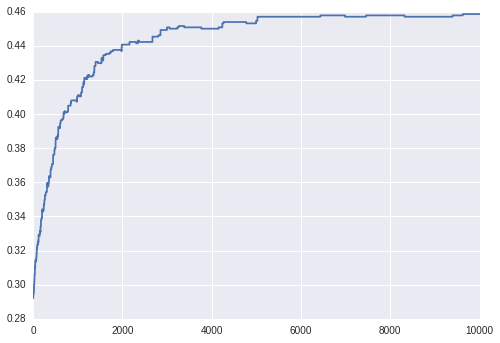# Schelling Model (basic)¶

TODO: Describe Schelling model and reference Think Complexity chapter.

### Imports¶

In this model, we'll be importing a few extra libraries that we haven't seen before:

In :
%matplotlib inline

# Imports
import copy
import itertools

import numpy
import matplotlib.pyplot as plt
import pandas

import seaborn; seaborn.set()

# Import widget methods
from IPython.html.widgets import *


## Building a grid¶

In the sample below, we'll create a simple square grid and fill the grid with households. The parameters below will guide our model as follows:

• grid_size: the number of cells per row or column; the total number of cells is $grid\_size^2$.
• group_proportion: the percentage of households that will be of type 1
• density: the percentage of grid cells that will be populated with a household

The logic for our grid initialization can be described as follows:

• For each cell in every row and column

• Draw a random value on $[0, 1)$ and compare to $density$ to determine if we will fill this cell
• If the cell will be filled, draw a random value on $[0, 1)$ and compare to $group\_proportion$ to determine whether the household will be 1 or 2
In :
# Set parameters
grid_size = 20
group_proportion = 0.25
density = 0.5

# Create the space and activate random cells
space = numpy.zeros((grid_size, grid_size), dtype=numpy.int8)

# Now sample the agents.
for row_id in range(grid_size):
for col_id in range(grid_size):
# Determine if this cell will be populated
if numpy.random.random() <= density:
# Determine this cell's initial group
if numpy.random.random() <= group_proportion:
cell_type = 1
else:
cell_type = 2

# Set the space
space[row_id, col_id] = cell_type

# Now show the space
f = plt.figure()
p = plt.pcolor(space, snap=True)
c = plt.colorbar()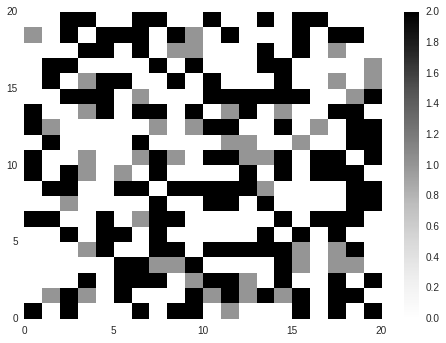### Initialization method¶

Below, we wrap the test method above in a method named initialize_space. We need to setup the following parameters:

• grid_size: number of cells in each row or column
• group_proportion: percentage of initial population that will be of group 1
• density: percentage of cells that will be occupied in the space
In :
def initialize_space(grid_size, group_proportion, density):
"""
Initialize a space.
"""

# Create the space and activate random cells
space = numpy.zeros((grid_size, grid_size), dtype=numpy.int8)

# Now sample the agents.
for row_id in range(grid_size):
for col_id in range(grid_size):
# Determine if this cell will be populated
if numpy.random.random() <= density:
# Determine this cell's initial group
if numpy.random.random() <= group_proportion:
cell_type = 1
else:
cell_type = 2

# Set the cell
space[row_id, col_id] = cell_type

return space


### Testing out space initialization¶

Let's test out our initialize_space method by visualizing for given parameters below.

In :
# Set parameters
grid_size = 10
group_proportion = 0.25
happy_proportion = 0.5
density = 0.5
window = 1

def display_space(grid_size=10, group_proportion=0.5, density=0.5):
# Check
assert(grid_size > 1)
assert(group_proportion >= 0.0)
assert(group_proportion <= 1.0)
assert(density >= 0.0)
assert(density <= 1.0)

# Initialize space
space = initialize_space(grid_size, group_proportion, density)

# Plot
f = plt.figure()
p = plt.pcolor(space)
c = plt.colorbar()

# Setup widget
interact(display_space,
grid_size=IntSliderWidget(min=2, max=100, value=10),
group_proportion=FloatSliderWidget(min=0.0, max=1.0, value=0.5),
density=FloatSliderWidget(min=0.0, max=1.0, value=0.5))

Out:
<function __main__.display_space>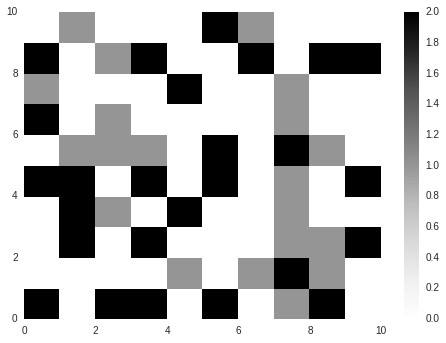In :
# Pick a random household
household_list = numpy.column_stack(numpy.where(space > 0))
household_id = numpy.random.choice(range(len(household_list)))

# Check if the household is happy
row, col = household_list[household_id]
household_type = space[row, col]

# Get the set of positions with grid wrapping for neighbors
neighbor_pos = [(x % grid_size, y % grid_size)
for x, y in itertools.product(range(row-window, row+window+1),
range(col-window, col+window+1))]
neighborhood = numpy.reshape([space[x, y] for x, y in neighbor_pos],
(2*window+1, 2*window+1))

# Count the number of neighbors of same type
neighbor_count = len(numpy.where(neighborhood == household_type)) - 1
neighbor_fraction = float(neighbor_count) / ((2 * window + 1) **2 - 1)

# Output counts
print("Household type: {0}".format(household_type))
print("Neighborhood:")
print(neighborhood)
print("Number of similar neighbors:")
print(neighbor_count)
print("Fraction of similar neighbors:")
print(neighbor_fraction)

Household type: 2
Neighborhood:
[[2 2 0]
[0 2 1]
[0 2 0]]
Number of similar neighbors:
3
Fraction of similar neighbors:
0.375

In :
def run_model_step(space, happy_proportion, window):
"""
Run  a step of the model.
"""
space = copy.copy(space)
grid_size = space.shape

# Get list of empty and occupied
household_list = numpy.column_stack(numpy.where(space > 0))

# Pick a random house
household_id = numpy.random.choice(range(len(household_list)))

# Check if the household is happy
row, col = household_list[household_id]
household_type = space[row, col]

# Get the set of positions with grid wrapping for neighbors
neighbor_pos = [(x % grid_size, y % grid_size)
for x, y in itertools.product(range(row-window, row+window+1),
range(col-window, col+window+1))]
neighborhood = numpy.reshape([space[x, y] for x, y in neighbor_pos],
(2*window+1, 2*window+1))

# Count the number of neighbors of same type
neighbor_count = len(numpy.where(neighborhood == household_type)) - 1
neighbor_fraction = float(neighbor_count) / ((2 * window + 1) **2 - 1)

# If the house is unhappy, move.
if neighbor_fraction < happy_proportion:
# Get empty cells
empty_list = numpy.column_stack(numpy.where(space == 0))

# Get empty target cell
target_cell_id = numpy.random.choice(range(len(empty_list)))
target_row, target_col = empty_list[target_cell_id]

# Move the agent
space[row, col] = 0
space[target_row, target_col] = household_type

return space

In :
# Set parameters
grid_size = 50
group_proportion = 0.33
happy_proportion = 0.33
density = 0.5
window = 3
max_steps = 100000

# Initialize space
space = initialize_space(grid_size, group_proportion, density)

# Setup space
space_history = [space]

# Iterate
for i in range(max_steps):
# Append step history
space_history.append(run_model_step(space_history[-1],
happy_proportion,
window))

In :
def display_space_step(step=1):
f = plt.figure()
plt.pcolor(space_history[step])
ax = f.gca()
ax.set_aspect(1./ax.get_data_ratio())

In :
interact(display_space_step,
step=IntSliderWidget(min=1, max=len(space_history)-1,
step=1))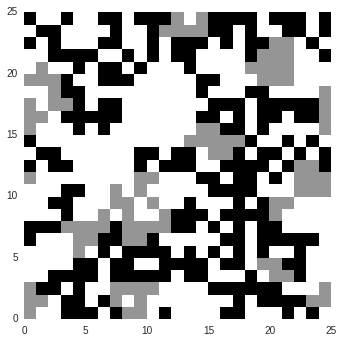### Automate simulation¶

In :
%%time

def run_model_simulation(grid_size = 50, group_proportion = 0.33, happy_proportion = 0.33,
density = 0.5, window = 1, max_steps = 100000):
"""
Run a full model simulation.
"""

# Initialize space
space = initialize_space(grid_size, group_proportion, density)

# Setup space
space_history = [space]

# Iterate
for i in range(max_steps):
# Append step history
space_history.append(run_model_step(space_history[-1],
happy_proportion,
window))

return space_history

# Run the simulation and output
space_history = run_model_simulation(grid_size=25, happy_proportion=0.25, window=1, max_steps=10000)
interact(display_space_step,
step=IntSliderWidget(min=1, max=len(space_history)-1,
step=1))In :
def get_neighbor_distribution(space, window=1):
"""
Get distribution of neighbor fractions.
"""

fractions = numpy.full(space.shape, numpy.nan)
grid_size = space.shape

# Get a measure of clustering
for row in range(grid_size):
for col in range(grid_size):
# Check if cell is occupied
if space[row, col] == 0:
continue
else:
household_type = space[row, col]

neighbor_pos = [1 for x, y in itertools.product(range(row-window, row+window+1),
range(col-window, col+window+1))
if space[x % grid_size, y % grid_size] == household_type]
fractions[row, col] = float(sum(neighbor_pos)-1) / ((2 * window + 1) **2 - 1)

return fractions

# Get the full happiness history
happy_history = []
happy_mean_ts = []
for step in range(len(space_history)):
happy_history.append(get_neighbor_distribution(space_history[step]))
happy_mean_ts.append(numpy.nanmean(happy_history[-1]))

In :
# Method to plot happiness surface
def display_happy_step(step=1):
# Create figures
f = plt.figure()
plt.pcolor(happy_history[step])
plt.colorbar()

# Create widget
interact(display_happy_step,
step=IntSliderWidget(min=1, max=len(happy_history)-1,
step=1))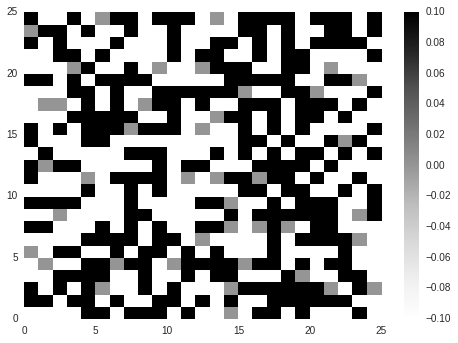In :
# Plot the average time series
f = plt.figure()
plt.plot(range(len(happy_mean_ts)), happy_mean_ts)

Out:
[<matplotlib.lines.Line2D at 0x8e21f510>]